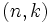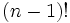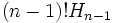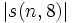# Unsigned Stirling number of the first kind

## Definition

The unsigned Stirling number of the first kind$|s(n,k)|$, also denoted$S_1(n,k)$ or$c(n,k)$, is defined as the number of elements in the symmetric group of degree$n$ whose cycle decomposition has exactly$k$ cycles. (Here, each fixed point is treated as a cycle of size one).

The unsigned Stirling numbers play a role in the probability distribution of number of cycles of permutations.

## General information

### Formulas in special cases

Case for$(n,k)$ Value of$|s(n,k)|$ Probability, i.e., value of$|s(n,k)|/n!$ Explanation$(n,1)$$(n - 1)!$$1/n$ must be a cyclic permutation with one cycle of size$n$, then use conjugacy class size formula for symmetric group.$(n,n)$$1$$1/n!$ can only be the identity permutation.$(n,n-1)$$\binom{n}{2} = \frac{n(n-1)}{2}$$\frac{1}{2[(n-2)!]}$ must be a transposition, determined by picking a subset of size two that gets transposed.$(n,2)$,$n$ odd$(n - 1)! H_{n-1}$, where$H_{n-1}$ is the harmonic number, i.e., the sum of reciprocals of first$n - 1$ natural numbers$H_{n-1}/n \approx (\log n)/n$

## Particular cases

Note that the unsigned Stirling numbers of the first kind for fixed$n$ form a unimodal (single-peaked) sequence in$k$, i.e., they are first increasing and then decreasing.$n$$n!$$|s(n,1)|$$|s(n,2)|$$|s(n,3)|$$|s(n,4)|$$|s(n,5)|$$|s(n,6)|$$|s(n,7)|$$|s(n,8)|$
1 1 1
2 2 1 1
3 6 2 3 1
4 24 6 11 6 1
5 120 24 50 35 10 1
6 720 120 274 225 85 15 1
7 5040 720 1764 1624 735 175 21 1
8 40320 5040 13068 13132 6769 1960 322 28 1